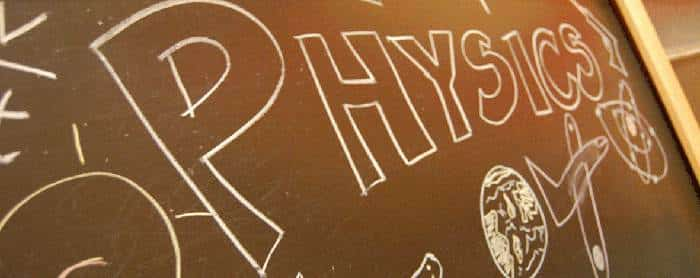Medical Sample papers

Total Internal Reflection QuestionsQuestions on Total Internal Reflection phenomenon (optics):

Ques: Finger prints on a piece of paper may be detected by sprinkling fluorescent powder on the paper and then looking it into
(a) Mercury light
(b) Sunlight
(c) Infrared light
(d) Ultraviolet light
Ans. (d)

Ques: The wavelength  of light in two liquids ‘x‘ and ‘y‘ is 3500 Å and 7000 Å, then the critical angle of x  relative to y will be
(a) 60°
(b) 45°
(c) 30°
(d) 15°
Ans. (c)

Ques: Relation between critical angles of water and glass is
(a) Cw > Cg
(b) Cw < Cg
(c) Cw = Cg
(d) Cw = Cg = 0
Ans. (a)

Ques: The reason for shining of air bubble in water is
(a) Diffraction of light
(b) Dispersion of light
(c) Scattering of light
(d) Total internal reflection of light
Ans. (d)

Ques: If critical angle for a material to air is 30o, the refractive index of the material will be
(a) 1.0
(b) 1.5
(c) 2.0
(d) 2.5
Ans. (c)

Related: MU OET Chemistry Mock Test

Ques: ‘Mirage’ is a phenomenon due to
(a) Reflection of light
(b) Refraction of light
(c) Total internal reflection of light
(d) Diffraction of light
Ans. (c)

Ques: The refractive index of water is 1.33. The direction in which a man under water should look to see the setting sun is
(a) 49o to the horizontal
(b) 90o with the vertical
(c) 49o to the vertical
(d) Along the horizontal
Ans. (c)

Ques: Optical fibres are related with
(a) Communication
(b) Light
(c) Computer
(d) None of these
Ans. (a)

Ques: The velocity of light in a medium is half its velocity in air.  If ray of light emerges from such a medium into air, the angle of incidence, at which it will be totally internally reflected, is
(a) 15o
(b) 30 o
(c) 45 o
(d) 60 o
Ans. (b)

Ques: Total internal reflection is possible when light rays travel
(a) Air to water
(b) Air to glass
(c) Glass to water
(d) Water to glass
Ans. (c)

Ques: Critical angle is that angle of incidence in the denser medium for which the angle of refraction in rarer medium is
(a) 0°
(b) 57°
(c) 90°
(d) 180°
Ans. (c)

Ques: A ray of light travelling in a transparent medium falls on a surface separating the medium from air at an angle of incidence of 45°. The ray undergoes total internal reflection. If n is the refractive index of the medium with respect to air, select the possible value (s) of n from the following
(a) 1.3
(b) 1.4
(c) 1.5
(d) 1.6
Ans. (c), (d)

Ques: When a ray of light emerges from a block of glass, the critical angle is
(a) Equal to the angle of reflection
(b) The angle between the refracted ray and the normal
(c) The angle of incidence for which the refracted ray travels along the glass-air boundary
(d) The angle of incidence
Ans. (c)

Related: Important MCQs on Plant Kingdom

Ques: The phenomenon utilised in an optical fibre is
(a) Refraction
(b) Interference
(c) Polarization
(d) Total internal reflection
Ans. (d)

Ques: Light wave enters from medium 1 to medium 2.  Its velocity in 2nd medium is double from 1st.  For total internal reflection the angle of incidence must be greater than
(a) 30o
(b) 60 o
(c) 45 o
(d) 90 o
Ans. (a)

Ques: Consider telecommunication through optical fibres.  Which of the following statements is not true
(a) Optical fibres may have homogeneous core with a suitable cladding
(b) Optical fibres can be of graded refractive index
(c) Optical fibres are subject to electromagnetic interference from outside
(d) Optical fibres have extremely low transmission loss
Ans. (c)

Ques: The phenomena of total internal reflection is seen when angle of incidence is
(a) 90o
(b) Greater than critical angle
(c) Equal to critical angle
(d) 0o
Ans. (b)

Ques: The critical angle of a medium with respect to air is . The refractive index of medium is
(a) 1.41
(b) 1.2
(c) 1.5
(d) 2
Ans. (a)

Ques: A normally incident ray reflected at an angle of 90o. The value of critical angle is
(a) 45o
(b) 90o
(c) 65o
(d) 43.2o
Ans. (b)